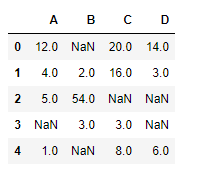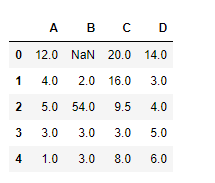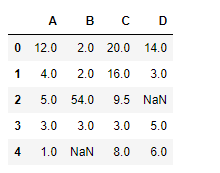Related Articles

# Python | Pandas dataframe.interpolate()

• Last Updated : 19 Nov, 2018

Python is a great language for doing data analysis, primarily because of the fantastic ecosystem of data-centric python packages. Pandas is one of those packages and makes importing and analyzing data much easier.

Pandas` dataframe.interpolate()` function is basically used to fill `NA` values in the dataframe or series. But, this is a very powerful function to fill the missing values. It uses various interpolation technique to fill the missing values rather than hard-coding the value.

Syntax: DataFrame.interpolate(method=’linear’, axis=0, limit=None, inplace=False, limit_direction=’forward’, limit_area=None, downcast=None, **kwargs)

Parameters :
method : {‘linear’, ‘time’, ‘index’, ‘values’, ‘nearest’, ‘zero’, ‘slinear’, ‘quadratic’, ‘cubic’, ‘barycentric’, ‘krogh’, ‘polynomial’, ‘spline’, ‘piecewise_polynomial’, ‘from_derivatives’, ‘pchip’, ‘akima’}

axis : 0 fill column-by-column and 1 fill row-by-row.
limit : Maximum number of consecutive NaNs to fill. Must be greater than 0.
limit_direction : {‘forward’, ‘backward’, ‘both’}, default ‘forward’
limit_area : None (default) no fill restriction. `inside `Only fill NaNs surrounded by valid values (interpolate). `outside `Only fill NaNs outside valid values (extrapolate). If limit is specified, consecutive NaNs will be filled in this direction.
inplace : Update the NDFrame in place if possible.
downcast : Downcast dtypes if possible.
kwargs : keyword arguments to pass on to the interpolating function.

Returns : Series or DataFrame of same shape interpolated at the NaNs

Example #1: Use `interpolate()` function to fill the missing values using linear method.

 `# importing pandas as pd``import` `pandas as pd`` ` `# Creating the dataframe ``df ``=` `pd.DataFrame({``"A"``:[``12``, ``4``, ``5``, ``None``, ``1``],``                   ``"B"``:[``None``, ``2``, ``54``, ``3``, ``None``],``                   ``"C"``:[``20``, ``16``, ``None``, ``3``, ``8``],``                   ``"D"``:[``14``, ``3``, ``None``, ``None``, ``6``]})`` ` `# Print the dataframe``df`Let’s interpolate the missing values using Linear method. Note that Linear method ignore the index and treat the values as equally spaced.

 `# to interpolate the missing values``df.interpolate(method ``=``'linear'``, limit_direction ``=``'forward'``)`

Output :As we can see the output, values in the first row could not get filled as the direction of filling of values is `forward` and there is no previous value which could have been used in interpolation.

Example #2: Use `interpolate()` function to interpolate the missing values in the backward direction using linear method and putting a limit on maximum number of consecutive `Na` values that could be filled.

 `# importing pandas as pd``import` `pandas as pd`` ` `# Creating the dataframe ``df ``=` `pd.DataFrame({``"A"``:[``12``, ``4``, ``5``, ``None``, ``1``],``                   ``"B"``:[``None``, ``2``, ``54``, ``3``, ``None``],``                   ``"C"``:[``20``, ``16``, ``None``, ``3``, ``8``],``                   ``"D"``:[``14``, ``3``, ``None``, ``None``, ``6``]})`` ` `# to interpolate the missing values``df.interpolate(method ``=``'linear'``, limit_direction ``=``'backward'``, limit ``=` `1``)`

Output :Notice the fourth column, only one missing value has been filled as we have put the limit to 1. The missing value in the last row could not get filled as no row exists after that from which the value could be interpolated.

Attention geek! Strengthen your foundations with the Python Programming Foundation Course and learn the basics.

To begin with, your interview preparations Enhance your Data Structures concepts with the Python DS Course. And to begin with your Machine Learning Journey, join the Machine Learning – Basic Level Course

My Personal Notes arrow_drop_up